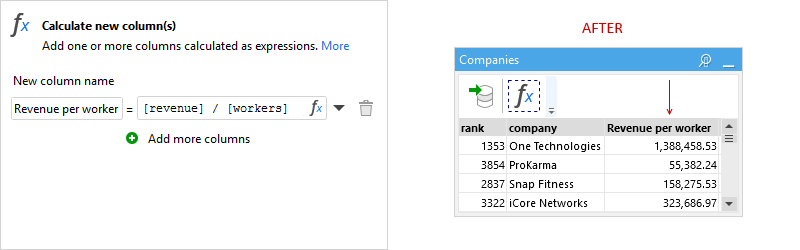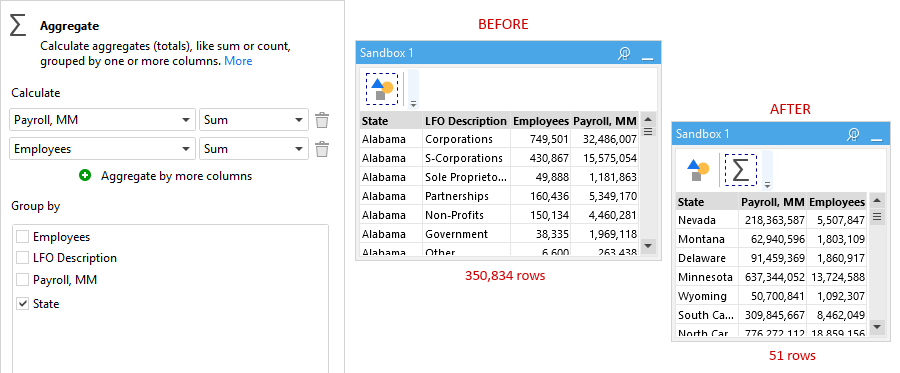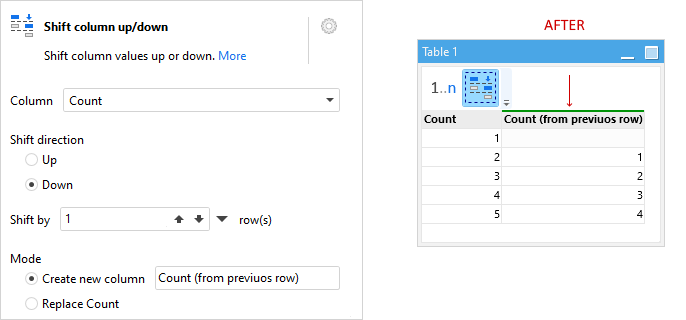## Calculations

### Calculate column

The Calculate new column action allows calculating new columns using expressions (i.e. formulas).Similarly, the Modify column action replaces existing columns. Note that it's possible to create/modify several columns in one action.

### Rule

The Rule action can be used for calculations that are nested IF / THEN / ELSE conditions. This action is similar to CASE statements in SQL. It can be used to create new columns as well as replace existing ones.### Aggregate

To calculate aggregates (grouped or not) such as sum or count, use the Aggregate action.